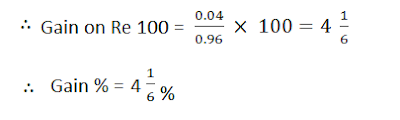# Profit and Loss Shortcuts - Using Rule of Fraction

The use of “Rule of Fraction” is dominant. We should understand this rule very well because it is going to be used in almost all the questions.### THE RULE OF FRACTION SAYS:

If our required value greater than the supplied value we should multiply the supplied value with a
fraction which is more than one. And if our required value is less than the supplied value we should multiply the supplied value with a fraction which is less than one.

• If there is a gain of x%, the calculating figure would be 100 and (100 + x).
• If there is loss of y%,the calculating figure would be 100 and (100 – x).
• If the required value is more than the supplied value, our multiplying  fractions   should be (100+x)/100  or 100/(100-y) ( both are greater than 1).
• If the required value is less than the supplied value, our multiplying fractions should be 100/(100+x)  or (100-y)/100   (both are less than 1).

Profit = selling price (SP) – cost price (CP)

Loss = Cost price (CP) – Selling price (SP)

### TO FIND THE GAIN OR LOSS PER CENT :-

The profit or loss is generally reckoned as so much per cent on the cost.

Gain or loss per cent = (Loss or gain)/CP  x 100

Ques - A man buys a toy for Rs 25 and sells it for Rs 30. Find his gain per cent.
Solution:  % gain = Gain/CP  x 100 = 5/25 x 100 = 20 %

Ques - A boy buys a pen for Rs 25 and sells it for Rs. 20. Find his loss per cent.
Solution:  % Loss = Loss/CP  x 100 = 5/25 x 100

Ques - If a man purchase 11 oranges for Rs. 10 and sells 10 oranges for Rs 11. How much profit or loss does he make?
Solution: Suppose that the person bought 11 x 10 = 110 oranges.
⇒ CP of 110 oranges = 10/11 x 110 = Rs 100
⇒ SP of 110 oranges = 11/10 x 110 = Rs 121

∴ Profit = Rs 121 – Rs 100 = Rs 21### QUICKER METHOD: REWRITE THE STATEMENT AS FOLLOWS:

Purchases            11 oranges for Rs 10

Sells                     10 oranges for Rs 11

Now, percentage profit or loss is given by:Since the sign is +ve, there is a gain of 21%

Ques - A man purchases 8 pens for Rs 9 and sells 9 pens for rupees 8. How much profit or loss does he make?
Solution:   quicker method:

Purchases            8 pens for Rs 9

Sells                     9 pens for Rs 8⇒ Since the sign is –ve, there is a loss of 20.98%

Ques - A dishonest dealer professes to sell his goods at cost price, but he uses a weight of 960 gm for the kg weight. Find his gain per cent.
Solution: Suppose goods cost the dealer Re 1 per kg. He sells for Rs 1 what cost him Rs. 0.96.
∴      Gain on Re 0.96 = Re 1 – Re 0.96 = Re 0.04### Must watch Video lecture on Profit and loss